### Creating Simple Turtle Shapes

Blocks Coding• Great for beginners
• Convert your code to text based coding at the end

Python coding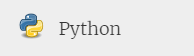• Learn to code like a pro
• You will make more errors when you first start, but eventually you will learn to code much faster!

Challenge 1 – Square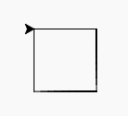• Draw a square with sides of 50 by 50

Challenge 2 – Rectangle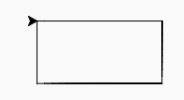• Draw a rectangle of sides 100 by 50

Challenge 3 – Equilateral Triangle• Draw an equilateral triangle of sides 90 long

Challenge 4 – Parallelogram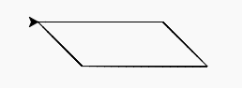• Create a parallelogram
• The length of sides 100 and 50
• The smallest internal angle should be 45 degrees

Challenge 5 – Zig Zag• Create a zigzagging line
• Each line part is 10 long
• The the turns are at 90 degrees
• It should zigzag 10 times

Challenge 6 – Star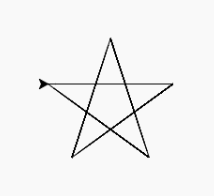• Create a 5 pointed star
• The length of each long line is 100

Challenge 7 – Circle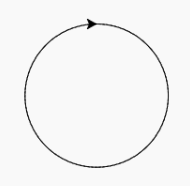• Draw a circle with a circumference of 360

Challenge 8 – Square Wave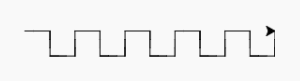• Create a square wave as above.
• Each length should be 20 long

Challenge 9 – Rounded Wave• Draw a rounded wave.
• First move to x location – 100
• circumference of a full circle would be 180